# Binomial theorem

In elementary algebra, the binomial theorem or the binomial expansion is a mechanism by which expressions of the form (x + y)n can be expanded. It is the identity that states that for any non-negative integer n,$(x + y)^n = \sum_{k=0}^n {n \choose k} x^k y^{n-k},$

where${n \choose k} = \frac{n!}{k!(n - k)!}$

is a binomial coefficient. Another useful way of stating it is the following: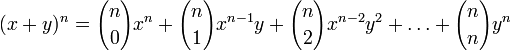$(x + y)^n = {n \choose 0} x^n + {n \choose 1} x^{n-1} y + {n \choose 2} x^{n-2} y^2 + \ldots + {n \choose n} y^n$

## Contents

### Pascal's triangle

An alternate way to find the binomial coefficients is by using Pascal's triange. The triangle is built from apex down, starting with the number one alone on a row. Each number is equal to the sum of the two numbers directly above it.

n=0         1
n=1        1 1
n=2       1 2 1
n=3      1 3 3 1
n=4     1 4 6 4 1
n=5   1 5 10 10 5 1


Thus, the binomial coefficients for the expression (x + y)4 are 1, 3, 6, 4, and 1.

## Proof

One way to prove this identity is by mathematical induction.

Base case: n = 0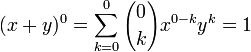$(x + y)^0 = \sum_{k=0}^0 {0 \choose k} x^{0-k} y^k = 1$

Induction case: Now suppose that it is true for n :$(x + y)^n = \sum_{k=0}^n {n \choose k} x^{n-k} y^k,$ and prove it for n + 1.$(x+y)^{n+1} = (x+y)(x+y)^n \,$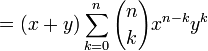$= (x+y) \sum_{k=0}^n {n \choose k} x^{n-k} y^k \,$$= \sum_{k=0}^n {n \choose k} x^{n+1-k} y^k + \sum_{j=0}^n {n \choose j} x^{n-j} y^{j+1} \,$$= \sum_{k=0}^n {n \choose k} x^{n+1-k} y^k + \sum_{j=0}^n {n \choose {(j+1) -1}} x^{n-j} y^{j+1} \,$$= \sum_{k=0}^n {n \choose k} x^{n+1-k} y^k + \sum_{k=1}^{n+1} {n \choose {k -1}} x^{n+1-k} y^k \,$$= \sum_{k=0}^{n+1} {n \choose k} x^{n+1-k} y^k - {n \choose {n+1}} x^0 y^{n+1}+ \sum_{k=0}^{n+1} {n \choose {k -1}} x^{n+1-k} y^k - {n \choose {-1}}x^{n+1} y^0 \,$$= \sum_{k=0}^{n+1} \left[ {n \choose k} + {n \choose {k -1}} \right] x^{n+1-k} y^k \,$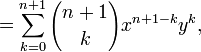$= \sum_{k=0}^{n+1} {{n+1} \choose k} x^{n+1-k} y^k,$

and the proof is complete.

## Examples

These are the expansions from 0 to 6.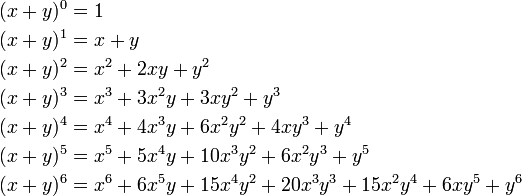\begin{align} (x + y)^0 &= 1 \\ (x + y)^1 &= x + y \\ (x + y)^2 &= x^2 + 2xy + y^2 \\ (x + y)^3 &= x^3 + 3x^2 y + 3xy^2 + y^3 \\ (x + y)^4 &= x^4 + 4x^3 y + 6x^2 y^2 + 4xy^3 + y^4 \\ (x + y)^5 &= x^5 + 5x^4 y + 10x^3 y^2 + 6x^2 y^3 + y^5 \\ (x + y)^6 &= x^6 + 6x^5 y + 15x^4 y^2 + 20x^3 y^3 + 15 x^2 y^4 + 6xy^5 + y^6 \end{align}

## Newton's binomial theorem

There is also Newton's binomial theorem, proved by Isaac Newton, that goes beyond elementary algebra into mathematical analysis, which expands the same sum (x + y)n as an infinite series when n is not an integer or is not positive.Some content on this page may previously have appeared on Citizendium.